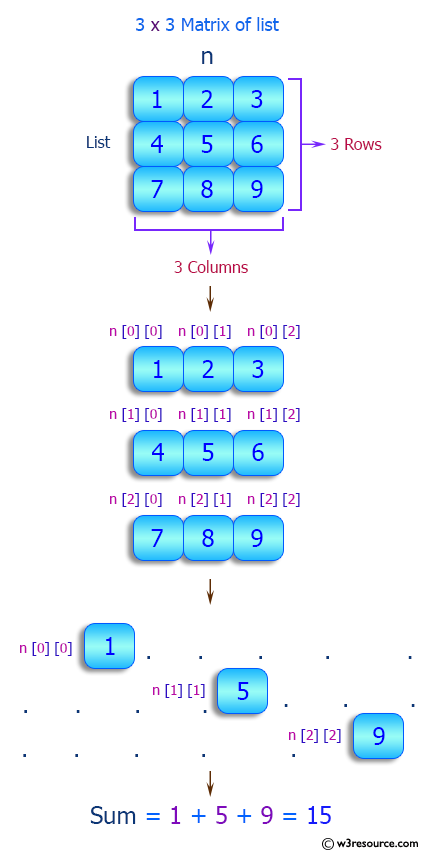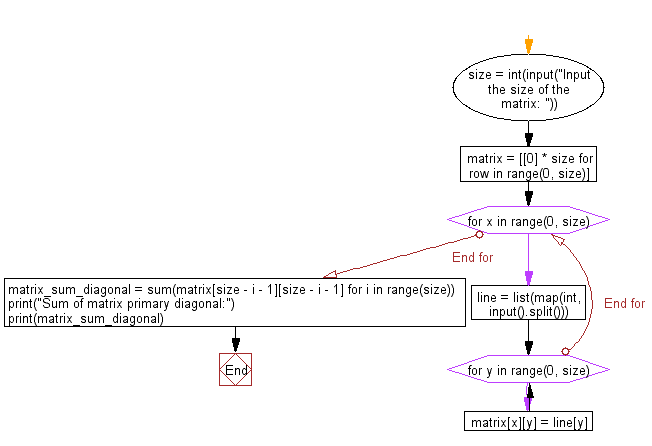﻿ Python: Read a square matrix from console and print the sum of matrix primary diagonal - w3resource# Python: Read a square matrix from console and print the sum of matrix primary diagonal

## Python List: Exercise - 88 with Solution

Write a Python program to read a square matrix from console and print the sum of matrix primary diagonal. Accept the size of the square matrix and elements for each column separated with a space (for every row) as input from the user.

Sample Solution:

Python Code:

``````size = int(input("Input the size of the matrix: "))
matrix = [ * size for row in range(0, size)]
for x in range(0, size):

line = list(map(int, input().split()))

for y in range(0, size):
matrix[x][y] = line[y]

matrix_sum_diagonal = sum(matrix[size - i - 1][size - i - 1] for i in range(size))
print("Sum of matrix primary diagonal:")
print(matrix_sum_diagonal)
```
```

Sample Output:

```Input the size of the matrix:  3
2 3 4
4 5 6
3 4 7
Sum of matrix primary diagonal:
14
```

Pictorial Presentation:Flowchart:## Visualize Python code execution:

The following tool visualize what the computer is doing step-by-step as it executes the said program:

Python Code Editor:

Have another way to solve this solution? Contribute your code (and comments) through Disqus.

What is the difficulty level of this exercise?

Test your Python skills with w3resource's quiz

﻿

## Python: Tips of the Day

```print(2_000_000)
```2000000